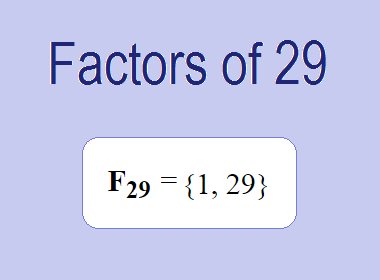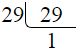# Factors of 29The factors of 29 are 1 and 29 i.e. F29 = {1, 29}. The factors of 29 are all the numbers that can divide 29 without leaving a remainder. 29 is a prime number, so it is divisible by 1 and 29 only.

We can check if these numbers are factors of 29 by dividing 29 by each of them. If the result is a whole number, then the number is a factor of 29. Let's do this for each of the numbers listed above:

·        1 is a factor of 29 because 29 divided by 1 is 29.

·        29 is a factor of 29 because 29 divided by 29 is 1.

## Properties of the Factors of 29

The factors of 29 have some interesting properties. One of the properties is that the sum of the factors of 29 is equal to 30. We can see this by adding all the factors of 29 together:

1 + 29 = 30

Another property of the factors of 29 is that the only prime factor of 29 is 29 itself.

********************

********************

## Applications of the Factors of 29

The factors of 29 have several applications in mathematics. One of the applications is in finding the highest common factor (HCF) of two or more numbers. The HCF is the largest factor that two or more numbers have in common. For example, to find the HCF of 29 and 58, we need to find the factors of both numbers and identify the largest factor they have in common. The factors of 29 are 1, and 29. The factors of 58 are 1, 2, 29, and 58. The largest factor that they have in common is 29. Therefore, the HCF of 29 and 58 is 29.

Another application of the factors of 29 is in prime factorization. Prime factorization is the process of expressing a number as the product of its prime factors. The prime factor of 29 is 29 since it is only the prime number that can divide 29 without leaving a remainder. Therefore, we can express 29 as:

29 = 29

We can do prime factorization by division method as given below,29 = 29

Since 29 is a prime number, there is no factor tree of 29.

## Conclusion

The factors of 29 are the numbers that can divide 29 without leaving a remainder. The factors of 29 are 1, and 29. The factors of 29 have some interesting properties, such as having a sum of 30. The factors of 29 have several applications in mathematics, such as finding the highest common factor and prime factorization.